• 主要介绍了Python实现Word表格转成Excel表格的示例代码，文中通过示例代码介绍的非常详细，对大家的学习或者工作具有一定的参考学习价值，需要的朋友们下面随着小编来一起学习学习吧
• 主要介绍了使用python处理题库表格并转化为word形式的实现,文中通过示例代码介绍的非常详细，对大家的学习或者工作具有一定的参考学习价值，需要的朋友们下面随着小编来一起学习学习吧
• 今日需求 其实就是把Word中的表格转到Excel中，顺便做一个调整。 如果单单是两个表格，那只要简单的复制黏贴即可，但如果上百了呢？那就得考虑自动化了。...path = "./word表格excel.docx" docx = Document
今日需求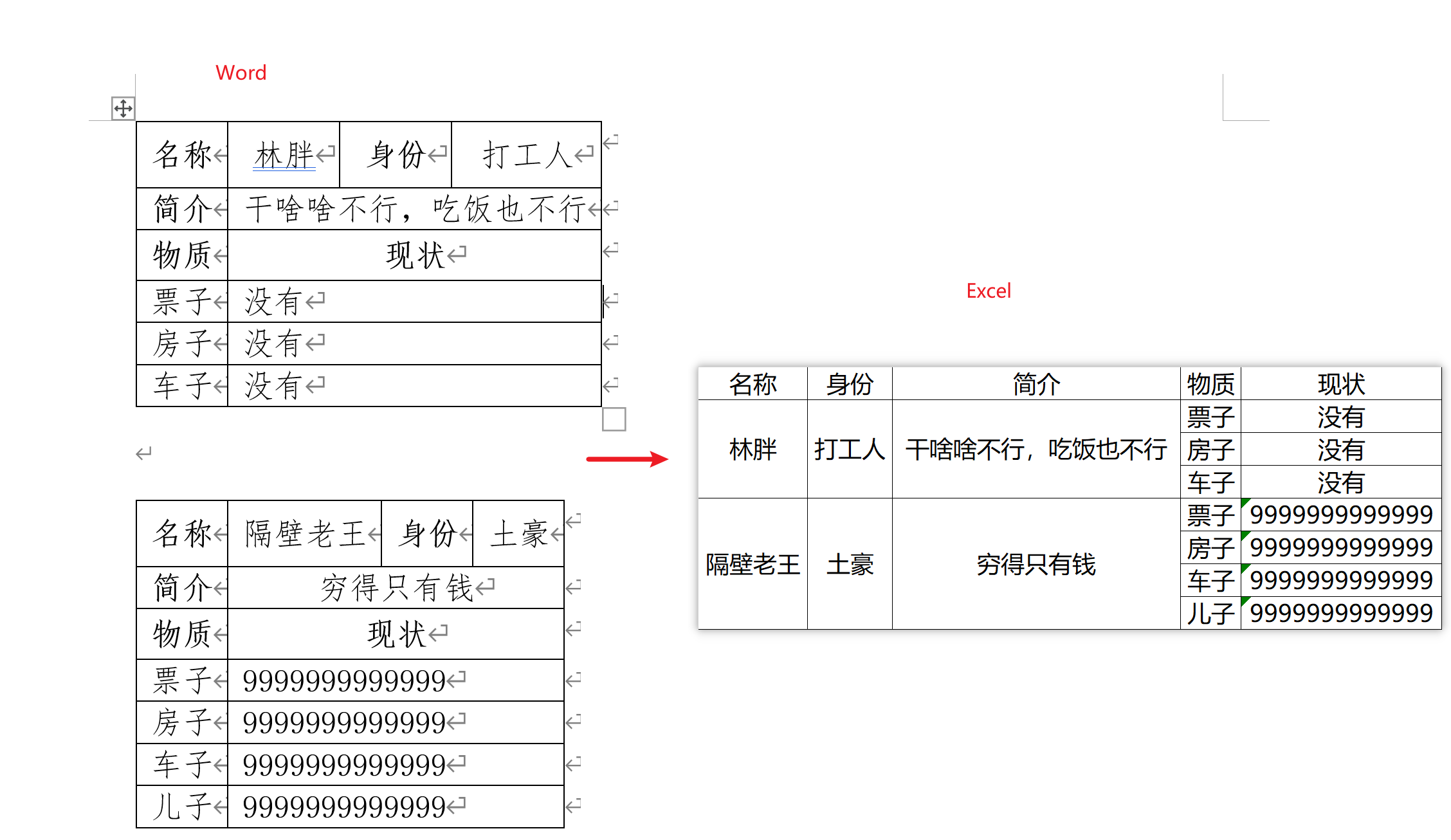其实就是把Word中的表格转到Excel中，顺便做一个调整。
如果单单是两个表格，那只要简单的复制黏贴即可，但如果上百了呢？那就得考虑自动化了。好在今天碰到的需求中的原文件格式是比较有规律的，那直接来尝试一下。
# 首先要pip install python-docx
# 如果原文件是doc格式，那就先转成docx
from docx import Document
import pandas as pd

path = "./word表格转excel.docx"
docx = Document(path)
table_s = docx.tables  # 返回一个Table对象的列表

len(table_s) --> 2 # 返回值是2，因为原文件中只有两个表格

list_ = []  # 初始化一个空列表，用来装后面的dict_

for table in table_s:  # 循环所有的表格列表
dict_ = {}
dict_['名称'] = table.cell(0, 1).text  # 表格的索引是从（0行，0列）开始
dict_['身份'] = table.cell(0, 3).text
dict_['简介'] = table.cell(1, 1).text
for i in range(3, len(table.rows)):  # 后续的内容格式不固定，所以循环获取
dict_[table.cell(i, 0).text] = table.cell(i, 1).text
list_.append(dict_)

当完成上述操作后,list_就会是一个字典列表。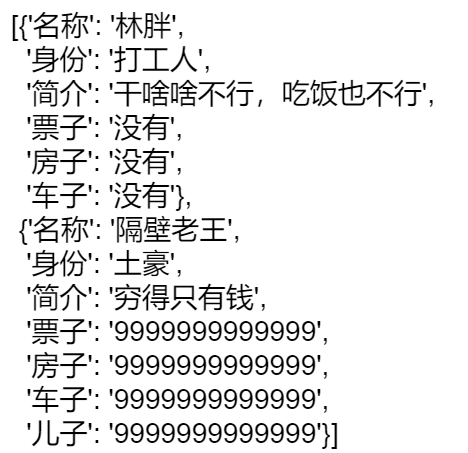接下来，将列表转为DataFrame进行操作即可。
df = pd.DataFrame(list_)

# 先将前三列作为索引，然后逆透视其他列，其实这就是个一维表转二维表的过程
df = df.set_index(['名称', '身份', '简介']).stack(level=0).to_frame()

# 重新设置一下行列索引的名字
df.index.names = ['名称', '身份', '简介', '物质']
df.columns = ['现状']

此时的df就跟需求结果相差不大了。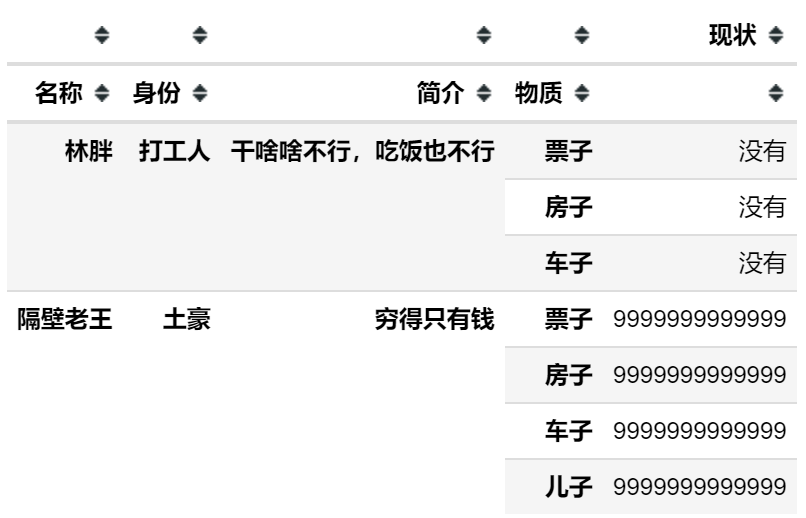最后将DataFrame导出，稍作美化即可完成需求。
# 导出的时候一定要设置index=True不然只会保留一列Series
df.to_excel("data3.xlsx", index=True, merge_cells=True)

完整代码
# 首先要pip install python-docx
# 如果原文件是doc格式，那就先转成docx
from docx import Document
import pandas as pd

path = "./word表格转excel.docx"
docx = Document(path)
table_s = docx.tables  # 返回一个Table对象的列表

list_ = []  # 初始化一个空列表，用来装后面的dict_

for table in table_s:  # 循环所有的表格列表
dict_ = {}
dict_['名称'] = table.cell(0, 1).text  # 表格的索引是从（0行，0列）开始的
dict_['身份'] = table.cell(0, 3).text
dict_['简介'] = table.cell(1, 1).text
for i in range(3, len(table.rows)):  # 循环获得后面的内容
dict_[table.cell(i, 0).text] = table.cell(i, 1).text
list_.append(dict_)

df = pd.DataFrame(list_)

# 先将前三列作为索引，然后逆透视其他列，就是个一维表转二维表的过程
df = df.set_index(['名称', '身份', '简介']).stack(level=0).to_frame()

# 重新设置一下行列索引的名字
df.index.names = ['名称', '身份', '简介', '物质']
df.columns = ['现状']

# 导出的时候一定要设置index=True不然只会保留一列Series
df.to_excel("data3.xlsx", index=True, merge_cells=True)

网盘链接 提取码：ujnx
展开全文• 想将一份 word 文件中的几个表格转写入 excel 文件中，后续用 excel 处理。 用到 python-docx 和 pandas 分别处理 wordexcel。 安装 python-docx：pip install python-docx，它不接受 .doc 文件，先转存为 .docx...
Notes
想将一份 word 文件中的几个表格转写入 excel 文件中，后续用 excel 处理。 用到 python-docx 和 pandas 分别处理 word 和 excel。 安装 python-docx：pip install python-docx，它不接受 .doc 文件，先转存为 .docx。 下面从 test.docx 读入，写到 test.xlsx。
code
from docx import Document
import pandas as pd

word = 'test.docx'
excel = 'test.xlsx'

doc = Document(word)  # word 文件
xls = pd.ExcelWriter(excel)  # 用来写入 excel

tables = doc.tables  # word 中所有 tables
# print(tables)
# tb = tables  # 第一个 table
# print(dir(tb))
# print(len(tb.rows), len(tb.columns))  # 行数、列数
for i, tb in enumerate(tables):
mat = []  # 用 list 套 list 的方法装二维表格内容
for r in range(1, len(tb.rows)):
row = []
for c in range(1, len(tb.columns)):
cell = tb.cell(r, c)
txt = cell.text if cell.text != '' else ' '  # 无内容用空格占位
row.append(txt)
mat.append(row)

# 用 mat 创建 DataFrame
df = pd.DataFrame(mat)
# print(df)
# DataFrame 写入 excel 中的某张 sheet

xls.save()  # 保存
xls.close()  # 关闭

References
python-docxpython读取word文档表格里的数据Pandas与EXCEL之数据读取/保存/文件分割/文件合并Python Pandas读取修改excel操作攻略python3 pandas读写excel
展开全文pandas
• # 只能处理列数一致的表格，不一致的请在word文档（转下行） # 中处理好后，再运行程序 ws['A1'] = '国家' ws['B1'] = '专利号' ws['C1'] = '自己设置' ws['D1'] = '懂？' ws['E1'] = '5' ws['F1'] = '6' ws['G1'] =...
准备工作
pip install docx pip install openpyxl
具体代码
# 没有的先pip install 包名称
from docx import Document
from openpyxl import Workbook

document = Document('Docx文件路径.dicx')

count = 0
tables = []
wb = Workbook()
ws = wb.active

# 设置列数，可以指定列名称，有几列就设置几个，
# A对应列1，B对应列2，以此类推
# 只能处理列数一致的表格，不一致的请在word文档（转下行）
# 中处理好后，再运行程序
ws['A1'] = '国家'
ws['B1'] = '专利号'
ws['C1'] = '自己设置'
ws['D1'] = '懂？'
ws['E1'] = '5'
ws['F1'] = '6'
ws['G1'] = '7'
ws['H1'] = '8'
ws['I1'] = '9'
ws['J1'] = '10'
ws['K1'] = '11'
ws['L1'] = '12'

total = len(document.tables)
print("总共", total, "个表格等待处理，请喝杯咖啡等待许久...")
for index in range(0, total):
table = []
for row in document.tables[index].rows:
line = []
for grid in row.cells:
line.append(grid.text)
table.append(line)
ws.append(line)
count = count + 1
print("第", count, "个表格正在处理...剩余", total - count + 1, "个表格", "\n")
tables.append(table)
# 测试专用，测试前30条数据请打开注释
# if count == 30:
#     break

wb.save("要保存xlsx的路径.xlsx")
print(tables)
print("表格处理完成...")


图片效果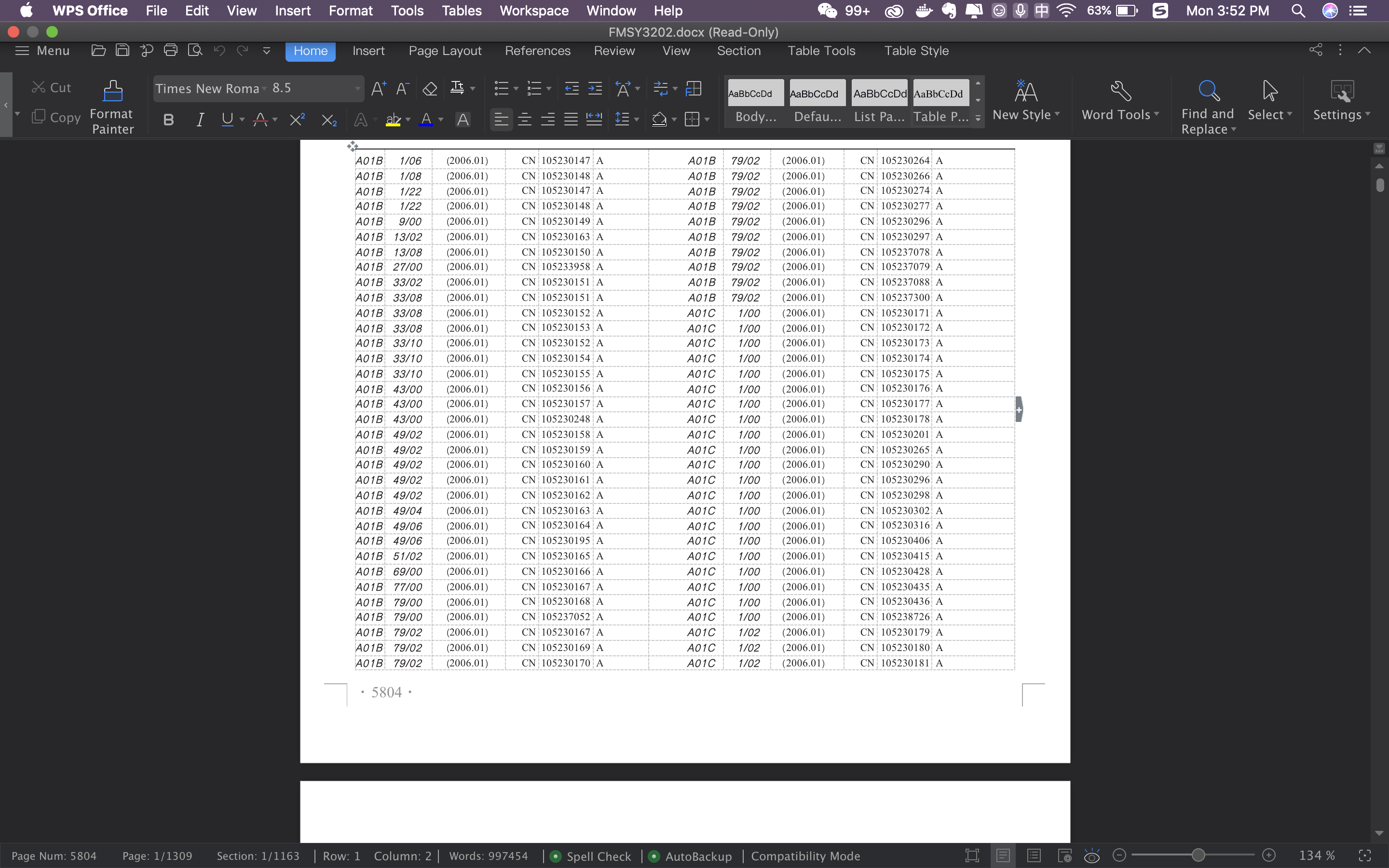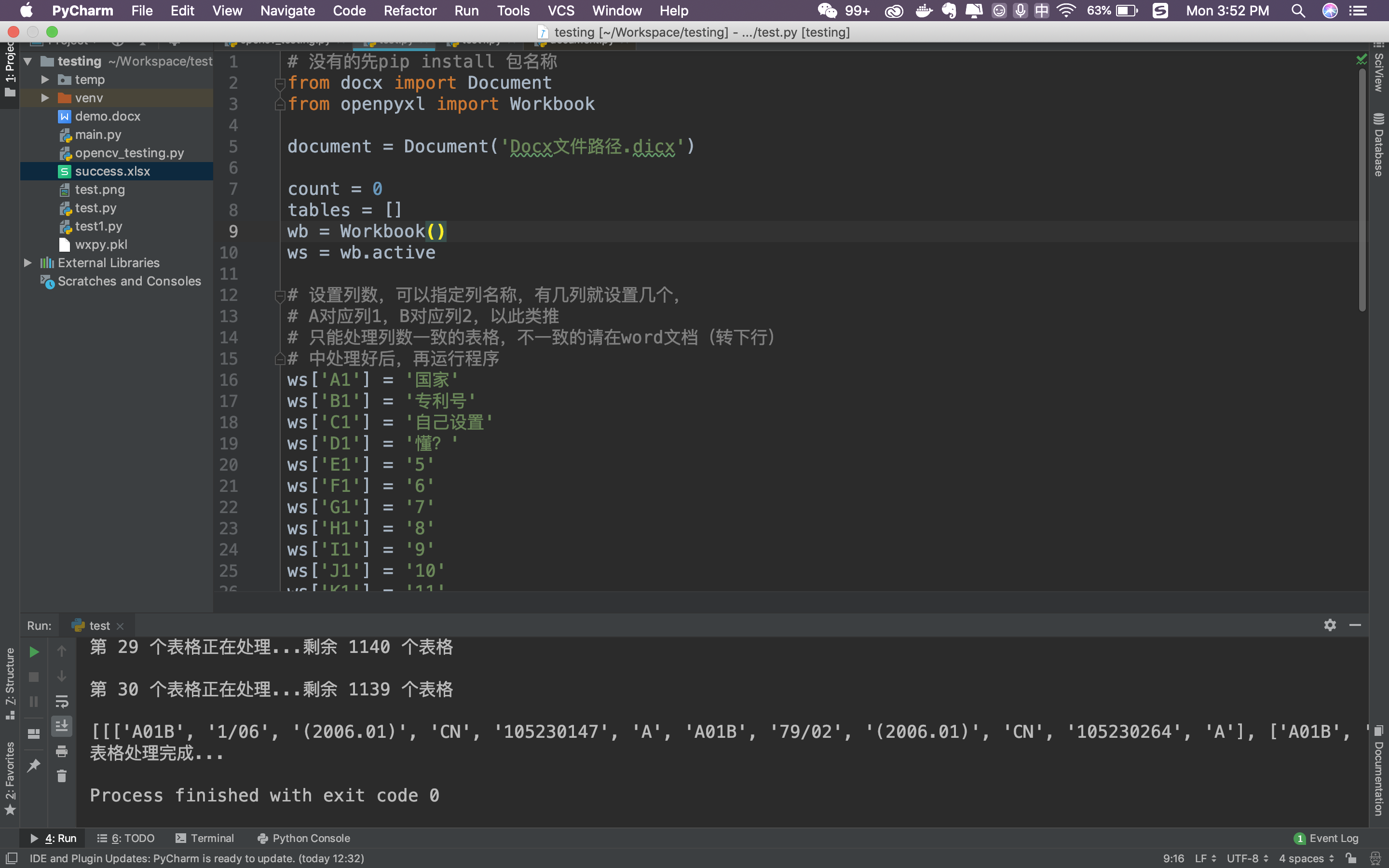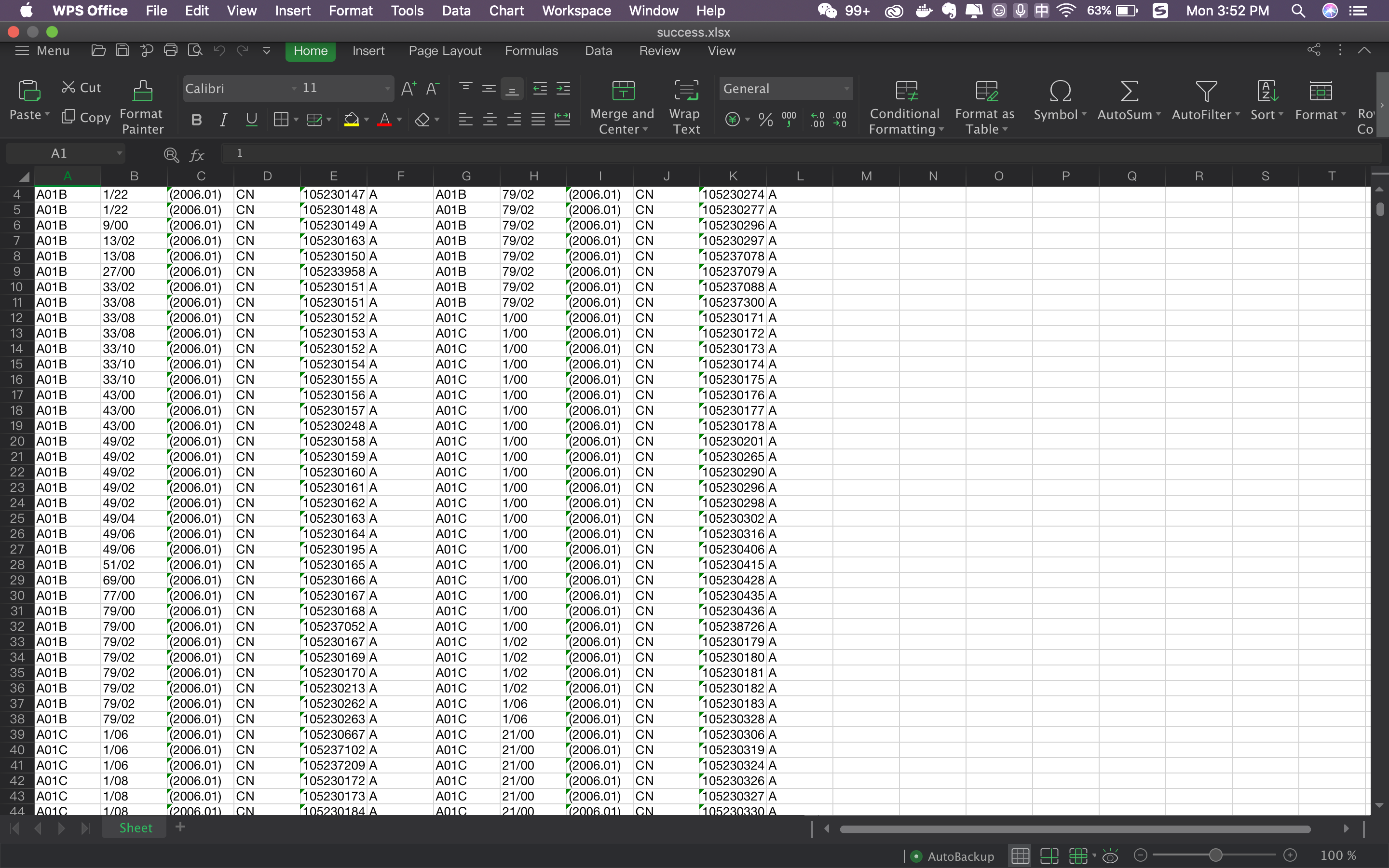附加说明
本文章主要以实现功能为主，相关优化工作请自行解决。 文中代码可能存在隐含异常，或者有更好的实现途径，也请读者自行解决，或者在评论区留言，当大家看到，分享你的意见。
谢谢，感谢阅读，更多文章扫码关注展开全文• 其实就是把Word中的表格转到Excel中，顺便做一个调整。这个需求在实际工作中，很多人还是经常碰到的！ 如果单单是两个表格，那只要简单的复制黏贴即可，但如果上百了呢？那就得考虑自动化了。好在今天碰到的需求中...
前言
本文的文字及图片来源于网络,仅供学习、交流使用,不具有任何商业用途,如有问题请及时联系我们以作处理。
PS：如有需要Python学习资料的小伙伴可以点击下方链接自行获取
Python免费学习资料、代码以及交流解答点击即可加入

今日需求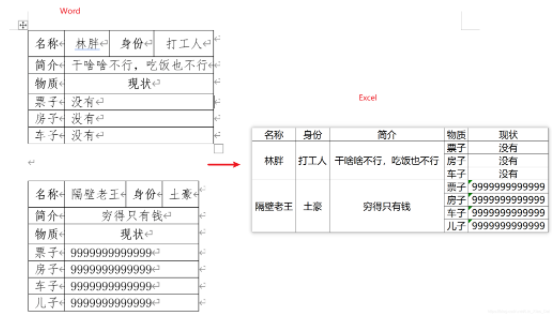其实就是把Word中的表格转到Excel中，顺便做一个调整。这个需求在实际工作中，很多人还是经常碰到的！
如果单单是两个表格，那只要简单的复制黏贴即可，但如果上百了呢？那就得考虑自动化了。好在今天碰到的需求中的原文件格式是比较有规律的，那直接来尝试一下。
# 首先要pip install python-docx
# 如果原文件是doc格式，那就先转成docx
from docx import Document
import pandas as pd

path = "./word表格转excel.docx"
docx = Document(path)
table_s = docx.tables  # 返回一个Table对象的列表

len(table_s) --> 2 # 返回值是2，因为原文件中只有两个表格

list_ = []  # 初始化一个空列表，用来装后面的dict_

for table in table_s:  # 循环所有的表格列表
dict_ = {}
dict_['名称'] = table.cell(0, 1).text  # 表格的索引是从（0行，0列）开始
dict_['身份'] = table.cell(0, 3).text
dict_['简介'] = table.cell(1, 1).text
for i in range(3, len(table.rows)):  # 后续的内容格式不固定，所以循环获取
dict_[table.cell(i, 0).text] = table.cell(i, 1).text
list_.append(dict_)

当完成上述操作后,list_就会是一个字典列表。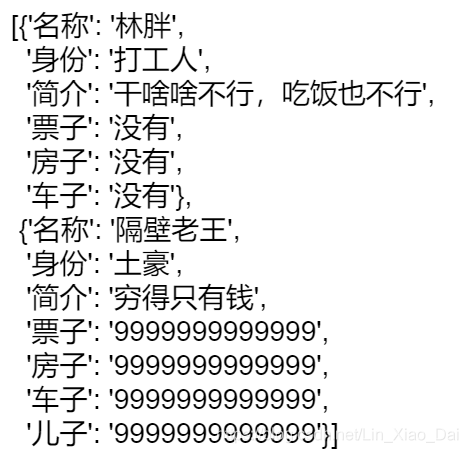接下来，将列表转为DataFrame进行操作即可。
df = pd.DataFrame(list_)

# 先将前三列作为索引，然后逆透视其他列，其实这就是个一维表转二维表的过程
df = df.set_index(['名称', '身份', '简介']).stack(level=0).to_frame()

# 重新设置一下行列索引的名字
df.index.names = ['名称', '身份', '简介', '物质']
df.columns = ['现状']

此时的df就跟需求结果相差不大了。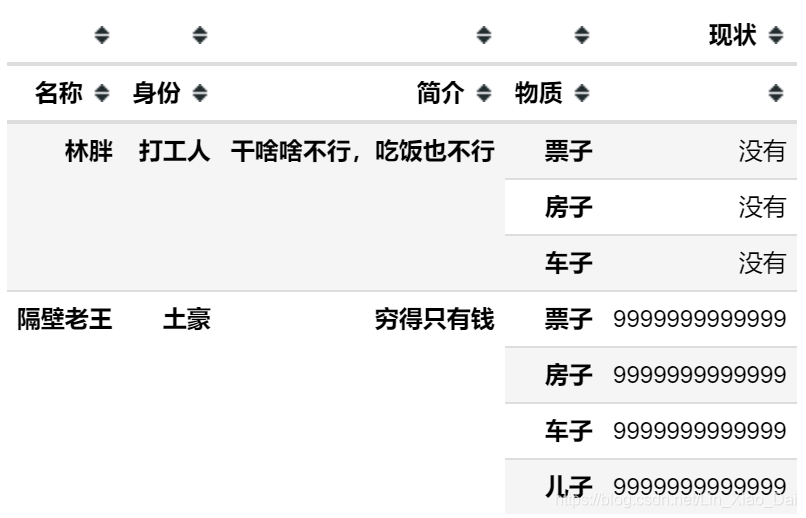最后将DataFrame导出，稍作美化即可完成需求。
# 导出的时候一定要设置index=True不然只会保留一列Series
df.to_excel("data3.xlsx", index=True, merge_cells=True)

完整代码
# 首先要pip install python-docx
# 如果原文件是doc格式，那就先转成docx
from docx import Document
import pandas as pd

path = "./word表格转excel.docx"
docx = Document(path)
table_s = docx.tables  # 返回一个Table对象的列表

list_ = []  # 初始化一个空列表，用来装后面的dict_

for table in table_s:  # 循环所有的表格列表
dict_ = {}
dict_['名称'] = table.cell(0, 1).text  # 表格的索引是从（0行，0列）开始的
dict_['身份'] = table.cell(0, 3).text
dict_['简介'] = table.cell(1, 1).text
for i in range(3, len(table.rows)):  # 循环获得后面的内容
dict_[table.cell(i, 0).text] = table.cell(i, 1).text
list_.append(dict_)

df = pd.DataFrame(list_)

# 先将前三列作为索引，然后逆透视其他列，就是个一维表转二维表的过程
df = df.set_index(['名称', '身份', '简介']).stack(level=0).to_frame()

# 重新设置一下行列索引的名字
df.index.names = ['名称', '身份', '简介', '物质']
df.columns = ['现状']

# 导出的时候一定要设置index=True不然只会保留一列Series
df.to_excel("data3.xlsx", index=True, merge_cells=True)
展开全文• 1.Word文档如下： 2.代码 # -*- coding: UTF-8 -*- from docx import Document def readSpecTable(filename, specText): document = Document(filename) paragraphs = document.paragraphs allTables = ...
• 通过Python自动化办公把提取word中的表格，填充到到excel当中. 首先通过for循环提取word当中的表格的每个单元格的内容，然后指定excel，进行批量填充
• 然后把信息更新到一个xls电子表格中，像下面这样（直接po结果好了） 而且点击文件路径可以直接打开对应的文件（含超链接） 代码实现 1. 采集docx里面文件头信息 # -*- coding:utf-8 -*- # 此程序可扫描
• 图片是Word的一种特殊内容，这篇文章主要介绍了关于Python操作word文档，向里面插入图片和表格的相关内容，下面话不多说了，来一起看看详细的代码 实例代码： # -*- coding: UTF8 -*- from docx import Document ...
• 这个写的很简单，比之前上传的那个vba的简单，逻辑如下，给定目录，遍历所有doc转换成docx，然后循环读取所有docx里的第一个表格，进行重复值筛选，然后写入到excel里，用到了几个模块，需要提前导入
• ## python 读取word表格，写入excel

千次阅读 热门讨论 2019-04-11 21:33:39
以上word表格汇总为下面的EXCEL表格 用到两个模块，docx,openpyxl 也可以写到csv文件，不过csv文件过长的数字文本会自动转科学计数 excel文件，内容没有转成数字，是默认插入的文本，如果加入判断语句又会有点...
• 一个基础的代码演示(并未对合并的单元格做处理), 可以读取Word文档中的所有表格/标题, 并在Excel中创建相应的sheet 希望能帮助到你. 原先的想法是可以直接打开一次execl然后一次性写入, 网上搜了一圈没有啥好办法, ...
• # 将excel表格装入itercars操作 itercars = iter(xl_sheet.rows) # 列名 hdr_cells = table.rows.cells for row in xl_sheet.rows: for num in range(0, excel_column): hdr_cells[num].text = u'' + str...
• 本文的文字及图片来源于网络,仅供学习、交流使用,...有一个下面这种形式的word表格： 希望能转换为下面这种格式的excel表格： 测试word文档读取 先测试一个word文档前1页的数据读取： from docx import ..数据分析
• 代码用于读Excel中的数据，然后批量写进word，文件的读写有专门的类，对于批量处理excel很有帮助
• doc文件转docx文件 根目录文件自动读取 批量处理word文件中指定关键词后的表格导入excel
• python快速将excel表格规范导入word前言二、开发环境三、实现步骤1.引入库2.代码片段 前言 最近接到一个需求，公司的统计需要一个excelword的工具，每天她都要人工复制粘贴excel中的表格Word合同中，而且粘贴后...pycharm 后端
• 在本篇文章里小编给大家整理的是关于python设置表格边框的具体方法及相关代码，需要的朋友们可以学习参考下。
• 点击上方“编程派”，选择设为“设为星标”优质文章，第一时间送达！大家好，今天有一个公务员的小伙伴委托我给他帮个忙，大概是有这样一份Word(由于涉及文件私密所以文中的具体内容已做修改)一...
• 亲人工作考试，公司给的题库好像是直接从数据库导出的表格Excel形式，在移动端上非常难看，需要不断左右上下滑动，看不了多少题眼就瞎了，遂主动请缨编写python脚本解决之。 原本给的题库在手机上横屏显示是这样的↓...
• WordExcel大概是我们最常碰到的两种文件了，我前面写过pandas的基本操作，利用它可以轻松完成Excel文件的批量处理，那么对于word文件的处理是否也有同样简单的方式，答案是肯定的，这涉及到python的另一个库docx，...docx 批处理
• python实现word转成自定义格式的excel文档（解决思路和代码） 支持按照文件夹去批量处理，也可以单独一个文件进行处理，并且可以自定义标识符 最近在开发一个答题类的小程序，到了录入试题进行测试的时候了，发现一...
• Python 读取excel及批量生成word模板 问题-来源 前几天，我姐遇见了一个难题：需要从excel表格中不断复制数据到word模板中，相当繁琐，询问我有没有解决的办法。于是我百度了一下，借鉴了相关博主的博文，结合自己的......

# python处理word表格excelpython 订阅